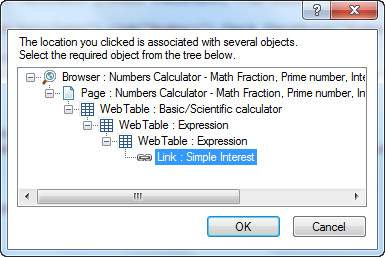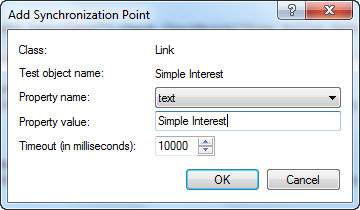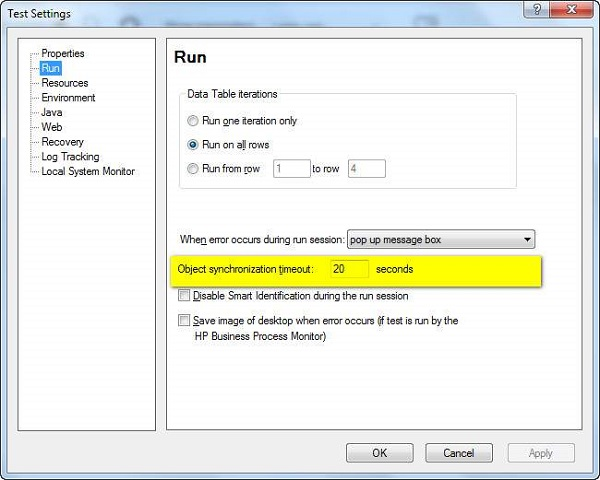# QTP - Synchronization

Synchronization point is the time interface between Tool and Application under test. Synchronization point is a feature to specify the delay time between two steps of the test script.

For example, clicking on a link may load the page is 1 second, sometimes 5 seconds or even it may take 10 seconds to load it completely. It depends on various factors such as the application-server response time, network bandwidth, and client system capabilities.

If the time is varying then the script will fail, unless the tester handles these time differences intelligently.

## Ways to Insert Sync Point

• WaitProperty
• Exist
• Wait
• Sync(only for web based apps)
• Inserting QTP Inbuilt Synchronization points.

Let us say, we need to insert a sync point between clicking on "numbers" link and clicking on "simple Interest" calculator in "www.easycalculation.com". We will now take a look at all the five ways to insert sync point for the above scenario.

### Method 1 − WaitProperty

WaitProperty is a method that takes the property name, Value and Timeout value as input to perform the sync. It is a dynamic wait and hence, this option is encouraged.

```' Method 1 - WaitProperty with 25 seconds
Dim obj
Set obj = Browser("Math Calculator").Page("Math Calculator")

```

### Method 2 − Exist

Exist is a method that takes the Timeout value as input to perform the sync. Again, it is a dynamic wait and hence this option is encouraged.

```' Method 2 : Exist Timeout - 30 Seconds
Dim obj
Set obj = Browser("Math Calculator").Page("Math Calculator")

Else
End IF
```

### Method 3 − Wait

Wait is a hardcoded sync point, which waits independent of the event happened or NOT. Hence, usage of Wait is discouraged and can be used for shorter wait time such as 1 or 2 seconds.

```' Method 3 : Wait Timeout - 30 Seconds
Dim obj
Set obj = Browser("Math Calculator").Page("Math Calculator")
wait(30)
```

### Method 4 − Sync Method

Sync Method can be used only for web applications where there is always a lag between page loads.

```' Method 4 :
Dim obj
Set obj = Browser("Math Calculator").Page("Math Calculator")

Browser("Math Calculator").Sync
```

### Method 5 − Inserting QTP Inbuilt Synchronization points

Step 1 − Get into Recording Mode. This option would be disabled if the user is NOT in Recording Mode.

Step 2 − Go to "Design" → "Synchronization Point".

Step 3 − We need to select the object, which we want to be the Sync Point. After selecting the object, object window opens as shown below −Step 4 − Click OK; the "Add Synchronization Window" opens. Select the Property, Value and Time out value and click OK as shown below −Step 5 − The script would be generated as shown below, which is the same as that of the WaitProperty(Method 1) that we had already discussed −

```Browser("Math Calculator").Page("Math Calculator").Link("Numbers").Click

Interest").WaitProperty "text", "Simple Interest", 10000
```

## Default Synchronization

When the user has not used any of the above sync methods, still QTP has an in-built Object synchronization timeout which can be adjusted by the user.

Navigate to "File" >> "Settings" >> Run Tab >> Object Synchronization Time out as shown below.